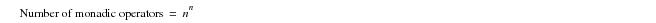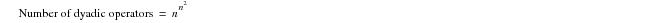# Number of Logical Operators Supported for Bivalent and Trivalent Logics - Teradata Database

Product
Release Number
15.10
Language
English (United States)
Last Update
2018-10-06
Product Category
Software

### Number of Logical Operators Supported for Bivalent and Trivalent Logics

In a bivalent logic, there are exactly four possible monadic, or single-operand, logical operators, as indicated in the following table. Note that the numberings have no meaning other than to distinguish the operators from one another.

 This operator number … Performs these mappings of TRUE and FALSE values … 1 T →  T F →  T 2 T →  F F →  F 3 T →  F F →  T 4 T →  T F →  F

By generalization, it is also true that there are exactly 16 possible dyadic, or two-operand, logical operators.

The formulas that produce the numbers of monadic and dyadic operators are provided as follows, where n represents the valence of the logic in question. For a bivalent logic, its value is 2; for a trivalent logic, its value is 3.By performing the calculations for a trivalent logic, you find that 3VLs have 27 possible monadic operators and 19,683 possible dyadic operators. These figures, of course, are redundant: they represent the number of possible operators, not the number of useful operators.

SQL, like most programming languages, overdetermines its operators. For example, the function performed by the = operator in the following two equations is not identical internally, but the language uses the same symbol for the operation in both cases.

• ‘value’ = ‘value’
• 2 = 2
• The issue raised here is whether SQL supports a sufficient number of trivalent logical operators to fully support a 3VL, and the answer to that question is negative.

Nevertheless, they indicate that SQL does not support anywhere near the number of logical operators required to support a coherent 3VL. Imagine the difficulty of trying to support the 4VL that Codd (1990) advocates, for which there is a possible 256 monadic operators and over four billion dyadic operators (4,294,967,296 to be exact). See Bolc and Borowik (1992, 2000) for examples of how complicated many‑valued logics are.